# SAT Math Multiple Choice Question 960: Answer and Explanation

### Test Information

Question: 960

12.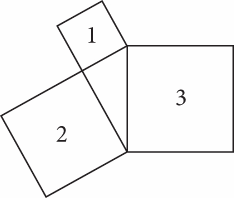Each of the quadrilaterals in the figure above is a square. The area of the smallestsquare (square 1) is 16 square units, and the area of the medium square (square 2)is 48 square units. What is the area, in square units, of the largest square (square3)?

• A. 56
• B. 64
• C. 78
• D. 96

Explanation:

B

Difficulty: Hard

Category: Additional Topics in Math / Geometry

Strategic Advice: Sometimes, working backward is necessary to solve a problem. Here, use the areas of the two smaller squares (squares 1 and 2) to find the side lengths, and then work in the opposite direction to find the area of the larger square (square 3).

Getting to the Answer: The area of a square is given by the formula A = s2. If the area of the smallest square is 16, then each side has length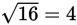. If the area of the medium square is 48, then a side has length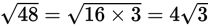. Use this information to label the diagram: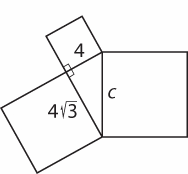Notice that the figure contained within the three squares is a right triangle with legs of lengths 4 and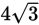. You might recognize these lengths as two pieces of the ratio of the sides for a 30-60-90 triangle, which is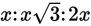. The hypotenuse of the triangle is given by 2x, so it has length 2(4) = 8. This means the side of the largest square has length 8, and its area is 82 = 64 square units, which is (B). (Note that you could also use the Pythagorean theorem to find the length of the hypotenuse of the triangle.)